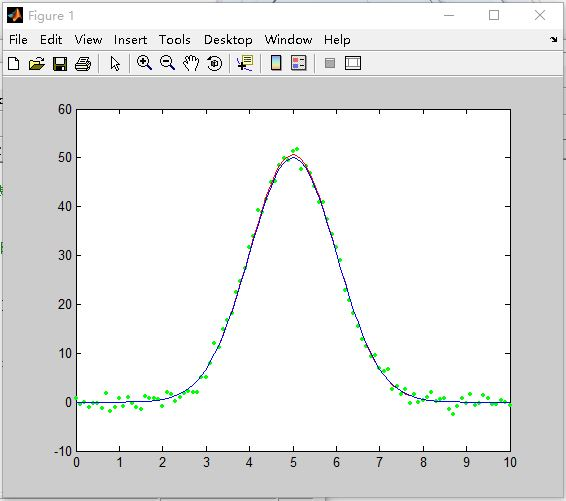• 完成某次课程任务，在MATLAB中新建一个m文件，将下列代码复制，或者直接复制到命令栏回车即可使用 x=[0:0.1:10];%0—10每次加0.1，共101个点 y=50*exp(-(x-5).^2./2);%x，y为初始函数值表现为红线 x=x';%因为拟合...
MATLAB实现高斯曲线拟合
完成某次课程任务，在MATLAB中新建一个m文件，将下列代码复制，或者直接复制到命令栏回车即可使用
x=[0:0.1:10];%0—10每次加0.1，共101个点
y=50*exp(-(x-5).^2./2);%x，y为初始函数值表现为红线
x=x';%因为拟合曲线时需要列向量
y=y';
y0=1*randn(1,101);%添加噪声//形成1行101列随机数
y0=y0';%y1为添加噪声后的函数，表现为点
y1=y+y0;%加上噪声后的函数值
Mytype=fittype('A*exp(-(x-u)^2/(2*d^2))');%需要拟合的函数类型
[cf ,gof]=fit(x(:),y1(:),Mytype)%fit函数
xi=0:0.1:10;
yi=cf.A*exp(-(xi-cf.u).^2/(2*cf.d^2));%xi，yi为拟合后的函数
plot(x,y1,'g.');%加噪声的函数为绿色的点
hold on;
plot(xi,yi,'r');%拟合后的曲线为红色的线
plot(x,y,'b');%拟合前的标准曲线为蓝色的线展开全文• 除了在Matlab中使用PRTools工具箱中的svm算法，Python中一样可以使用支持向量机做分类。因为Python中的sklearn库也集成了SVM算法，本文的运行环境是Pycharm。一、导入sklearn算法包　Scikit-Learn库已经实现了所有...
除了在Matlab中使用PRTools工具箱中的svm算法，Python中一样可以使用支持向量机做分类。因为Python中的sklearn库也集成了SVM算法，本文的运行环境是Pycharm。一、导入sklearn算法包　　Scikit-Learn库已经实现了所有基本机器学习的算法，具体使用详见官方文档说明：http://scikit-learn.org/stable/auto_examples/index.html#support-vector-machines。　　skleran中集成了许多算法，其导入包的方式如下所示，　　逻辑回归：from sklearn.linear_model import LogisticRegression  朴素贝叶斯：from sklearn.naive_bayes import GaussianNBK-近邻：from sklearn.neighbors import KNeighborsClassifier　　决策树：from sklearn.tree import DecisionTreeClassifier　　支持向量机：from sklearn import svm二、sklearn中svc的使用(1)使用numpy中的loadtxt读入数据文件　　loadtxt()的使用方法：　　　　fname:文件路径。eg：C:/Dataset/iris.txt。　　dtype：数据类型。eg：float、str等。　　delimiter：分隔符。eg：‘，’。　　converters：将数据列与转换函数进行映射的字典。eg：{1:fun}，含义是将第2列对应转换函数进行转换。　　usecols：选取数据的列。　　以Iris兰花数据集为例子：　　由于从UCI数据库中下载的Iris原始数据集的样子是这样的，前四列为特征列，第五列为类别列，分别有三种类别Iris-setosa， Iris-versicolor， Iris-virginica。　　　　　　　当使用numpy中的loadtxt函数导入该数据集时，假设数据类型dtype为浮点型，但是很明显第五列的数据类型并不是浮点型。　　因此我们要额外做一个工作，即通过loadtxt()函数中的converters参数将第五列通过转换函数映射成浮点类型的数据。　　首先，我们要写出一个转换函数：123def iris_type(s):it = {'Iris-setosa': 0, 'Iris-versicolor': 1, 'Iris-virginica': 2}return it[s]　　接下来读入数据，converters={4: iris_type}中“4”指的是第5列：12path = u'D:/f盘/python/学习/iris.data'  # 数据文件路径data = np.loadtxt(path, dtype=float, delimiter=',', converters={4: iris_type})　　读入结果：　　(2)将Iris分为训练集与测试集123x, y = np.split(data, (4,), axis=1)x = x[:, :2]x_train, x_test, y_train, y_test = train_test_split(x, y, random_state=1, train_size=0.6)　　1. split(数据，分割位置，轴=1(水平分割) or 0(垂直分割))。　　2. x = x[:, :2]是为方便后期画图更直观，故只取了前两列特征值向量训练。　　3. sklearn.model_selection.train_test_split随机划分训练集与测试集。train_test_split(train_data,train_target,test_size=数字, random_state=0)　　参数解释：　　train_data：所要划分的样本特征集　　train_target：所要划分的样本结果　　test_size：样本占比，如果是整数的话就是样本的数量　　random_state：是随机数的种子。　　随机数种子：其实就是该组随机数的编号，在需要重复试验的时候，保证得到一组一样的随机数。比如你每次都填1，其他参数一样的情况下你得到的随机数组是一样的。但填0或不填，每次都会不一样。随机数的产生取决于种子，随机数和种子之间的关系遵从以下两个规则：种子不同，产生不同的随机数；种子相同，即使实例不同也产生相同的随机数。(3)训练svm分类器123clf = svm.SVC(C=0.1, kernel='linear', decision_function_shape='ovr')clf = svm.SVC(C=0.8, kernel='rbf', gamma=20, decision_function_shape='ovr')clf.fit(x_train, y_train.ravel())　　kernel='linear'时，为线性核，C越大分类效果越好，但有可能会过拟合(defaul C=1)。　　 kernel='rbf'时(default)，为高斯核，gamma值越小，分类界面越连续；gamma值越大，分类界面越“散”，分类效果越好，但有可能会过拟合。　　decision_function_shape='ovr'时，为one v rest，即一个类别与其他类别进行划分，　　decision_function_shape='ovo'时，为one v one，即将类别两两之间进行划分，用二分类的方法模拟多分类的结果。(4)计算svc分类器的准确率123456print clf.score(x_train, y_train)  # 精度y_hat = clf.predict(x_train)show_accuracy(y_hat, y_train, '训练集')print clf.score(x_test, y_test)y_hat = clf.predict(x_test)show_accuracy(y_hat, y_test, '测试集') 结果为：　　如果想查看决策函数，可以通过decision_function()实现12print 'decision_function:n', clf.decision_function(x_train)print 'npredict:n', clf.predict(x_train) 结果为：　　decision_function中每一列的值代表距离各类别的距离。(5)绘制图像　　1.确定坐标轴范围，x，y轴分别表示两个特征12345x1_min, x1_max = x[:, 0].min(), x[:, 0].max()  # 第0列的范围x2_min, x2_max = x[:, 1].min(), x[:, 1].max()  # 第1列的范围x1, x2 = np.mgrid[x1_min:x1_max:200j, x2_min:x2_max:200j]  # 生成网格采样点grid_test = np.stack((x1.flat, x2.flat), axis=1)  # 测试点print 'grid_test = n', grid_testgrid_hat = clf.predict(grid_test)       # 预测分类值grid_hat = grid_hat.reshape(x1.shape)  # 使之与输入的形状相同　　这里用到了mgrid()函数，该函数的作用这里简单介绍一下：　　 假设假设目标函数F(x，y)=x+y。x轴范围1~3，y轴范围4~6，当绘制图像时主要分四步进行：　　【step1：x扩展】(朝右扩展)：   [1 1 1][2 2 2]　　 [3 3 3]　　【step2：y扩展】(朝下扩展)：　　 [4 5 6]　　 [4 5 6]　　 [4 5 6]　　【step3：定位(xi，yi)】：　　 [(1,4) (1,5) (1,6)]　　 [(2,4) (2,5) (2,6)]　　 [(3,4) (3,5) (3,6)]　　【step4：将(xi，yi)代入F(x,y)=x+y】　　因此这里x1, x2 = np.mgrid[x1_min:x1_max:200j, x2_min:x2_max:200j]后的结果为：　　　　再通过stack()函数，axis=1，生成测试点　　　　2.指定默认字体12mpl.rcParams['font.sans-serif'] = [u'SimHei']mpl.rcParams['axes.unicode_minus'] = False　　3.绘制123456789101112cm_light = mpl.colors.ListedColormap(['#A0FFA0', '#FFA0A0', '#A0A0FF'])cm_dark = mpl.colors.ListedColormap(['g', 'r', 'b'])plt.pcolormesh(x1, x2, grid_hat, cmap=cm_light)plt.scatter(x[:, 0], x[:, 1], c=y, edgecolors='k', s=50, cmap=cm_dark)  # 样本plt.scatter(x_test[:, 0], x_test[:, 1], s=120, facecolors='none', zorder=10)  # 圈中测试集样本plt.xlabel(u'花萼长度', fontsize=13)plt.ylabel(u'花萼宽度', fontsize=13)plt.xlim(x1_min, x1_max)plt.ylim(x2_min, x2_max)plt.title(u'鸢尾花SVM二特征分类', fontsize=15)plt.grid()plt.show() 　　pcolormesh(x,y,z,cmap)这里参数代入x1，x2，grid_hat，cmap=cm_light绘制的是背景。　　 scatter中edgecolors是指描绘点的边缘色彩，s指描绘点的大小，cmap指点的颜色。　　 xlim指图的边界
展开全文• 背景在数字图像高斯噪声的主要来源出现在采集期间。 由于不良照明和或高温引起的传感器噪声。所谓高斯噪声是指概率密度函数服从高斯分布（即正态分布）的一类噪声。高斯分布高斯分布，也称正态分布，又称常态...
背景在数字图像中的高斯噪声的主要来源出现在采集期间。 由于不良照明和或高温引起的传感器噪声。所谓高斯噪声是指概率密度函数服从高斯分布（即正态分布）的一类噪声。高斯分布高斯分布，也称正态分布，又称常态分布，记为，其中为分布的参数，分别为高斯分布的期望和方差。当有确定值时，p(x)也就确定了，特别当时，的分布为标准正态分布。matlab代码matlab代码也很简单,函数也有很多,最常见的是randn()函数sigma = 0.1;
mu = 0.001;
noise = mu+sigma*randn([3,3]);图像实例clc
clear all
close all

%%
% img = double(imread('wagon.jpg'))/255;
% img = double(imread('strawberries.jpg'))/255;
img = double(imread('onion.png'))/255;
max(img(:))
min(img(:))

%%
dim_img = size(img)
sigma = 0.1
noise = sigma.*randn(dim_img);
img_noise = img + noise;
max(noise(:))
min(noise(:))
%%
figure,
subplot(1,3,1)
imshow(img)
subplot(1,3,2)
imshow(img_noise)
subplot(1,3,3)
imshow(noise)均值为0方差为0.1的gauss noise但是这样添加噪声之后有一个缺点,噪声的取值有正有负,在图片的表示中,小于0的一律为黑色,因此噪音图中黑色才会占大部分.参考^高斯噪声 https://baike.baidu.com/item/%E9%AB%98%E6%96%AF%E5%99%AA%E5%A3%B0^matlab中的randn函数 https://ww2.mathworks.cn/help/matlab/ref/randn.html?searchHighlight=randn&s_tid=srchtitle^imnoise函数添加椒盐噪声 https://zhuanlan.zhihu.com/p/117591137
展开全文• matlab拟合数据有工具箱cftool (支持多项式 指数 高斯多种函数拟合.)  当x-y有对应关系时，即x,y都是已知数据集,用 polyfit函数 可以作多项式拟合 polyfit(x,y,n) n表示多项式次数  实际往往自变量和因变量 有...
    最近要用matlab处理一批数据 ，作非线性拟合。matlab拟合数据有工具箱cftool (支持多项式 指数  高斯多种函数拟合.)
当x-y有对应关系时，即x,y都是已知数据集,用 polyfit函数  可以作多项式拟合 polyfit(x,y,n)  n表示多项式次数
实际中往往自变量和因变量 有参数待定  需要用模型反求待定参数， 使得在此模型下，得到最优参数，则可以尝试nlinfit
nlinfit支持多个自变量   y=f(x1,x2,----)

自定义拟合函数
function rs=nonfit(w,r)
%w为待定系数  r为传入自变量x ，這里有 五个自变量  y=f(x1,x2,x3,x4,x5)
rs=calKBS(w(1).*r(:,1)+w(2).*r(:,2)+w(3).*r(:,3)+w(4).*r(:,4)+(1-w(1)-w(2)-w(3)-w(4)).*r(:,5))./w(5);  end
%  函数调用    w0为初始值
result= nlinfit(x(:,:,20);,density,@nonfit,w0);

附  squeeze函数可以去除矩阵多余维数  如 3*1*6*1*7维度数据 变为3*6*7

展开全文• 1、拟合参数精度不够，导致拟合曲线计算的输出值与原函数值相差较大，比如高斯拟合时峰值位置偏移较大。 这时我们可将拟合的模型保存到工作空间（save xxfit to workspace），在命令窗口输入‘coeffvalues(xxfit ...
• 背景在数字图像高斯噪声的主要来源出现在采集期间。 由于不良照明和或高温引起的传感器噪声。所谓高斯噪声是指概率密度函数服从高斯分布（即正态分布）的一类噪声。高斯分布高斯分布，也称正态分布，又称常态...
• 而化学分析，有许多具有明确物理意义的二维谱图，图光谱、色谱等，其信号峰位置、峰高、峰宽等均具有实际物理意义，因此，提出使用高斯曲线进行数据拟合和表征。 高斯函数仅适用于对称谱图，其简图如下： 假设...信号分析
• 时间：2017-03-16 20:30:20声明：欢迎被转载，记得注明出处~前言本文为曲线与分布拟合的一部分，主要介绍正态分布、拉普拉斯分布等常用分布拟合的理论推导以及代码实现。一、理论推导假设数据独立同分布。对于任意...
• %%%三维平面拟合 %%%生成随机数据 %内点 mu=[0 0 0]; %均值 S=[2 0 4;0 4 0;4 0 8]; %协方差 data1=mvnrnd(mu,S,300); %产生200个高斯分布数据 %外点 mu=[2 2 2]; S=[8 1 4;1 8 2;4 2 8]; %协方差 data2=...
• 1.确定要拟合的类型 　一般情况下matlab会直接提供常用的类型，用fittype创建拟合模型。至于matlab具体提供了哪些模型，参见帮助"List ... %高斯拟合 　如果库没有自己想要的拟合形式，可以自己进行定义，此时...
• ## matlab拟合工具简介

千次阅读 2014-09-28 09:08:26
matlab命令行里输入cftool命令可以打开图形化曲线拟合工具箱，能够提供多项式函数、指数函数、高斯函数、正弦函数、幂函数等多种形式的拟合。用户只需要输入拟合数据、指定函数形式，工具箱就可以自动计算出拟合...数据
• 基于qt creator开发环境下的高斯曲线拟合实现过程： 空气VOCs色谱图得到的一系列离散数据，色谱峰...Matlab中具有强大的矩阵运算功能，容易实现。本文软件在qt creator下实现则调用了Eigen矩阵库，进行矩阵操作。 ...
• 正态分布（Normal distribution）又名高斯分布（Gaussian distribution），是一个在数学、物理及工程等领域都非常重要的概率分布，在统计学的许多方面有着重大的影响力。 若随机变量X服从一个数学期望为μ、标准...
• 有时候的实验室数据图像得到的如下图所示：当然之前中过冷水多次有跟大家提多项式拟合、傅里叶级数拟合高斯级数拟合，实际更加常见的操作是用matlab中图像拟合工具箱cftool灵活进行函数拟合。这个过程大多数读者...
• 该程序包通过期望最大化（EM）算法拟合高斯混合模型（GMM），适用于任意维度的数据集。 应用了多种技术来提高数值稳定性，例如在对数域计算概率以避免在计算高维数据概率时经常发生的浮点数下溢。 该代码还通过...
• 对此，提出，可以通过高斯尺度空间对背景进行估计，得到大致背景图像，再用原图减去估计的背景，得到目标图像，通过伽马校正突出目标图像后，最后使用高斯分布拟合优化的Otsu算法进行全局分割，得到...
• 最小二乘法拟合椭圆——MATLAB和Qt-C++实现 https://blog.csdn.net/sinat_21107433/article/details/80877758 以上文章，C++代码有问题。因此参考如下文章，得到正确的结果。 矩阵求逆-高斯消元法介绍及其实现 ...椭圆 java android
• 最初我试图拟合一个多项式方程z=a+bx+cx^2+dy+exy+fy^2)然后计算高斯曲率$K = \frac{F_{xx}\cdot F_{yy}-{F_{xy}}^2}{(1+{F_x}^2+{F_y}^2)^2}$但是，如果曲面是复杂的，则问题是拟合的。我找到了这个Matlab程序来...
• ## Matlab学习小记

千次阅读 2011-01-28 13:18:00
大部分文章所写的公式和Matlab里面的高斯公式相同，再加上一个断面有两个方向的开挖，所以，拟合曲线就应该是两个高斯公式叠加（至少我是这么认为的，也没问导师。于是，我决定在Matlab的环境下来编写这个程序，以前...sql server jdbc microsoft sqlserver
• % MATLAB数学建模工具箱 % % 本工具箱主要包含三部分内容 % 1. MATLAB常用数学建模工具的中文帮助 % 2. 贡献MATLAB数学建模工具(打*号) % 3. 中国大学生数学建模竞赛历年试题MATLAB程序 % 数据拟合 % interp1 - ...
• ## MATLAB常用算法

热门讨论 2010-04-05 10:34:28
各种数学算法的MATLAB实现 第4章： 插值 函数名 功能 Language 求已知数据点的拉格朗日插值多项式 Atken 求已知数据点的艾特肯插值多项式 Newton 求已知数据点的均差形式的牛顿插值多项式 Newtonforward 求已知数据...
• % matlab11\toolbox\mathmodl增至path,放在最前面，并保存设置； % 3. 回到你的工作目录。现在MATHMODL已成为一个普通的工具箱了。 % % 可以使用命令help mathmodl查看内容或直接用命令mathmodl学习教程。 % % ...
• % matlab11\toolbox\mathmodl增至path,放在最前面，并保存设置； % 3. 回到你的工作目录。现在MATHMODL已成为一个普通的工具箱了。 % % 可以使用命令help mathmodl查看内容或直接用命令mathmodl学习教程。 % % ...
• 1.熟悉Matlab内置线性规划函数的使用 2.对Iris数据集中的数据求取协方差和相关性系数矩阵。并对该数据集做KL变换。 3.随机产生100组数据，每组数据有25个点，数据点为函数sin(2*pi*x)加上高斯噪声，使用Ridge回归对...
• 这个模型的设计思路是基于构建一系列的用于衡量图像质量的特征，并且将这些特征用于拟合一个多元的高斯模型，这些特征是从一些简单并且高度规则的自然景观提取；这个模型实际上是衡量一张待测图像在多元分布上的...
• MATLAB语言常用算法程序集 书4-17章代码，都是一些常用的程序 第4章： 插值 函数名 功能 Language 求已知数据点的拉格朗日插值多项式 Atken 求已知数据点的艾特肯插值多项式 Newton 求已知数据点的均差形式的牛顿...
• RANSAC在图像拼接有所使用，有时候也在图像理解的相关算法有所使用。 算法简介如下(摘自《图像处理、分析与机器视觉（第3版）》)： 1.假设我们要将n个数据点X={...3.从X随机选取m个项并拟合一个模型。（我这...# matlab中高斯拟合matlab 订阅